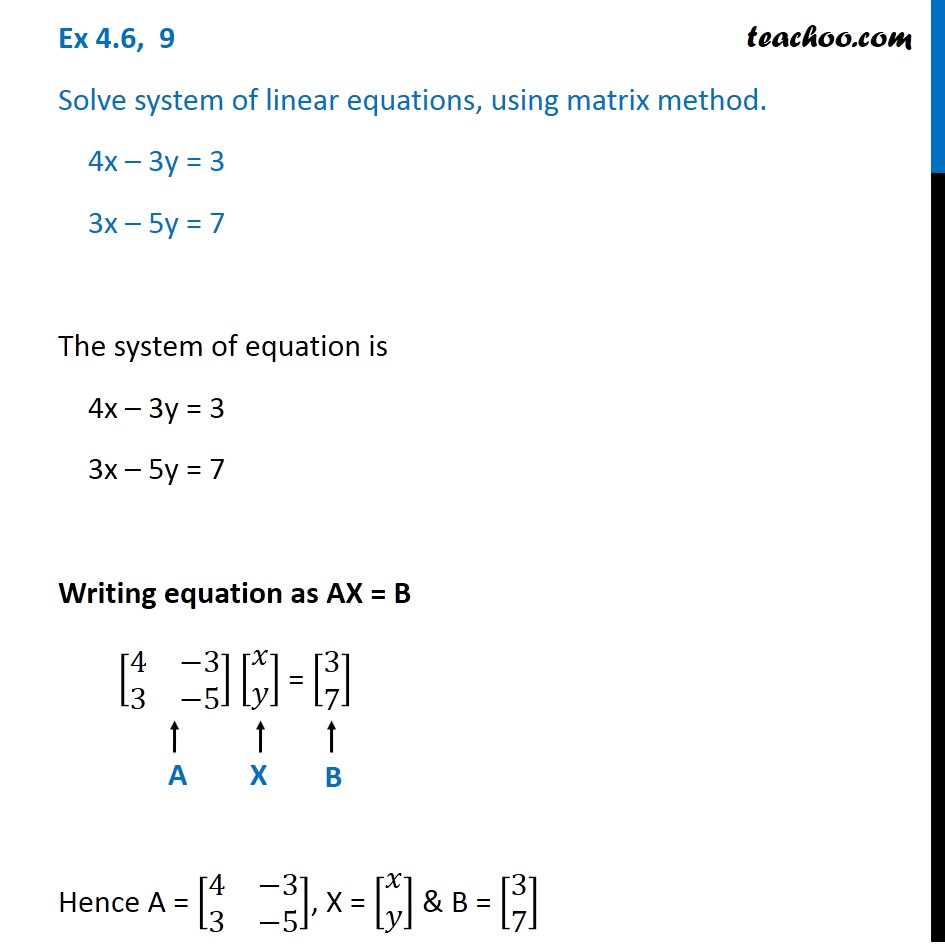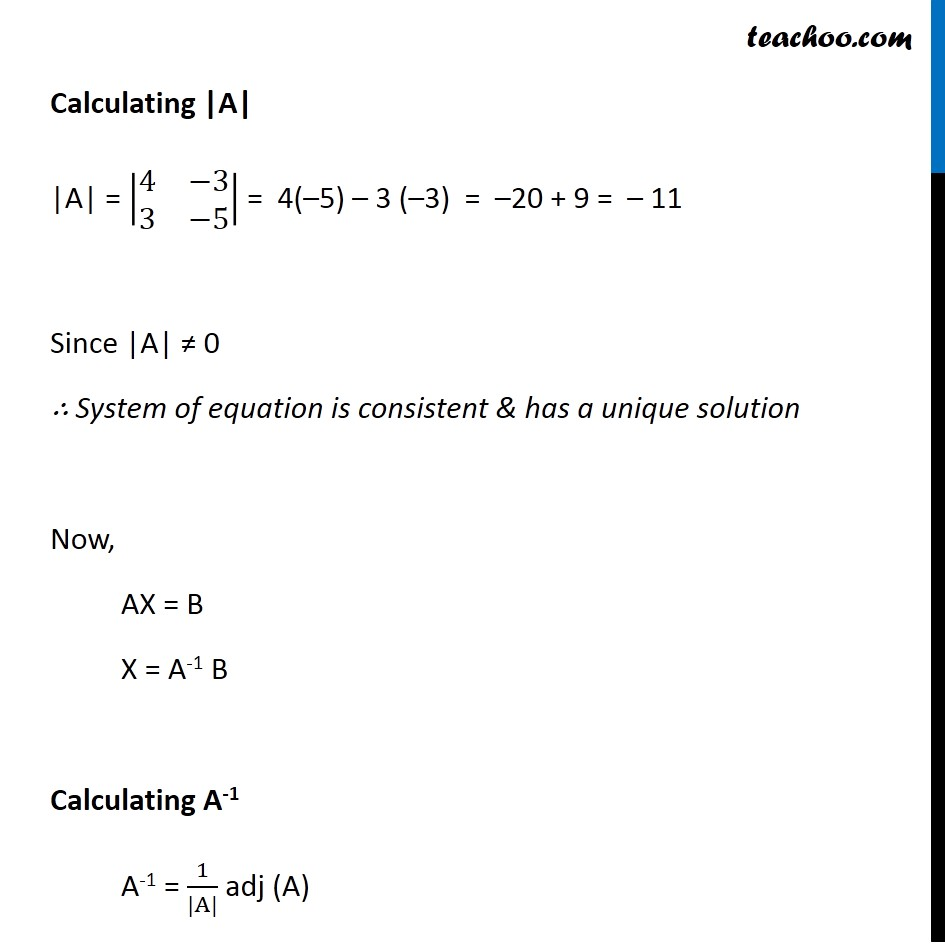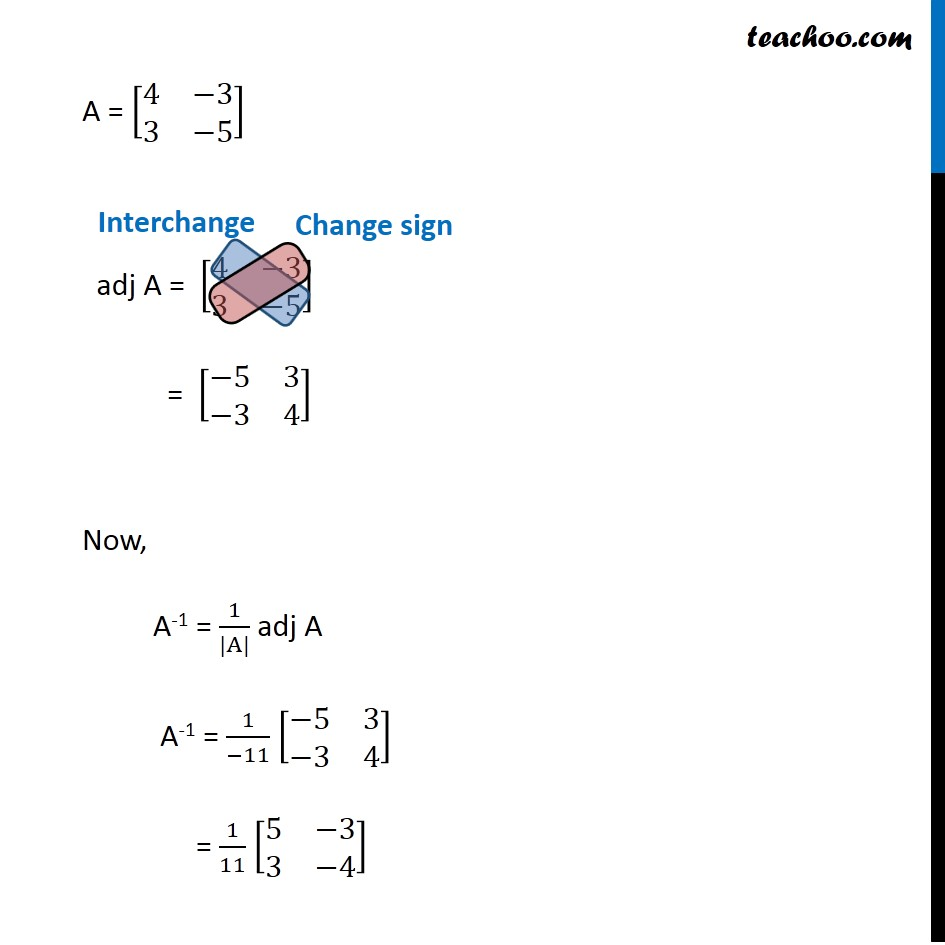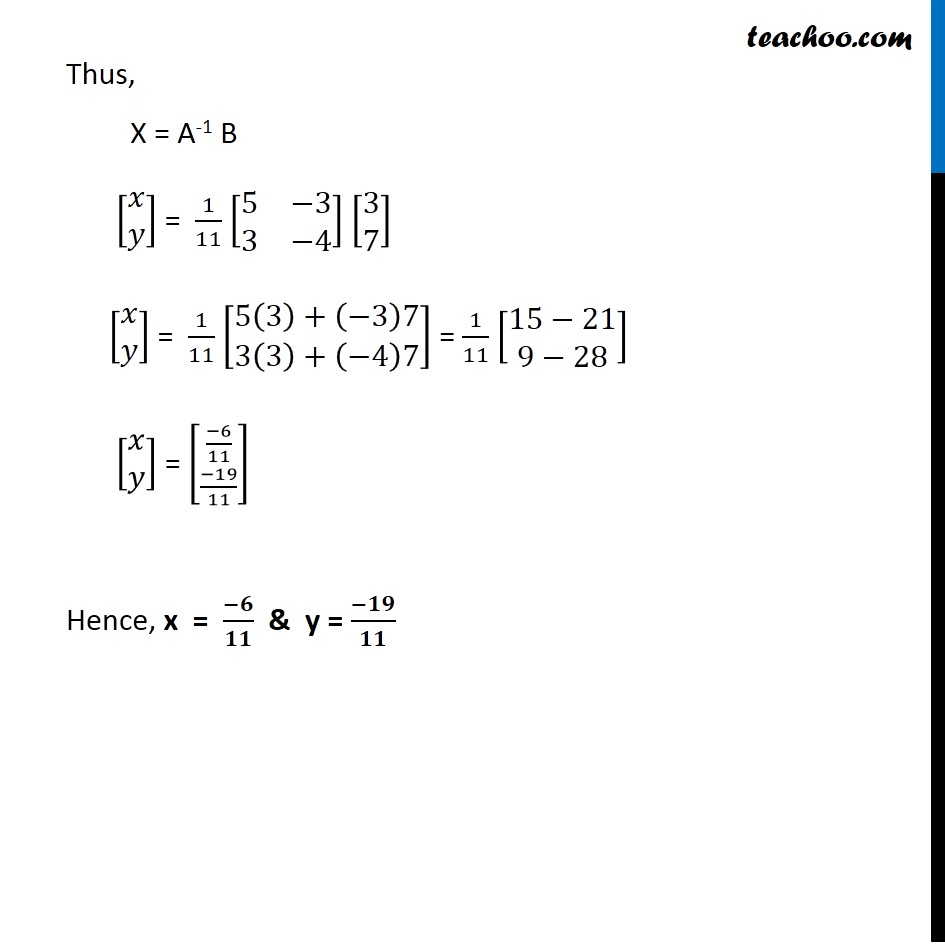Find solution of equations- Equations given

Chapter 4 Class 12 Determinants
Concept wiseLearn in your speed, with individual attention - Teachoo Maths 1-on-1 Class

### Transcript

Ex 4.5, 9 Solve system of linear equations, using matrix method. 4x – 3y = 3 3x – 5y = 7 The system of equation is 4x – 3y = 3 3x – 5y = 7 Writing equation as AX = B [■8(4&−[email protected]&−5)] [■8(𝑥@𝑦)] = [■8([email protected])] Hence A = [■8(4&−[email protected]&−5)], X = [■8(𝑥@𝑦)] & B = [■8([email protected])] Calculating |A| |A| = |■8(4&−[email protected]&−5)| = 4(–5) – 3 (–3) = –20 + 9 = – 11 Since |A| ≠ 0 ∴ System of equation is consistent & has a unique solution Now, AX = B X = A-1 B Calculating A-1 A-1 = 1/(|A|) adj (A) A = [■8(4&−[email protected]&−5)] adj A = [■8(4&−[email protected]&−5)] = [■8(−5&3@−3&4)] Now, A-1 = 1/(|A|) adj A A-1 = 1/(−11) [■8(−5&3@−3&4)] = 1/11 [■8(5&−[email protected]&−4)] Thus, X = A-1 B [■8(𝑥@𝑦)] = 1/11 [■8(5&−[email protected]&−4)] [■8([email protected])] [■8(𝑥@𝑦)] = 1/11 [■8(5(3)+(⤶7−3)[email protected](3)+(−4)7)] = 1/11 [■8(15−[email protected]−28)] [■8(𝑥@𝑦)] = [((−6)/11)¦((−19)/11)] Hence, x = (−𝟔)/𝟏𝟏 & y = (−𝟏𝟗)/𝟏𝟏